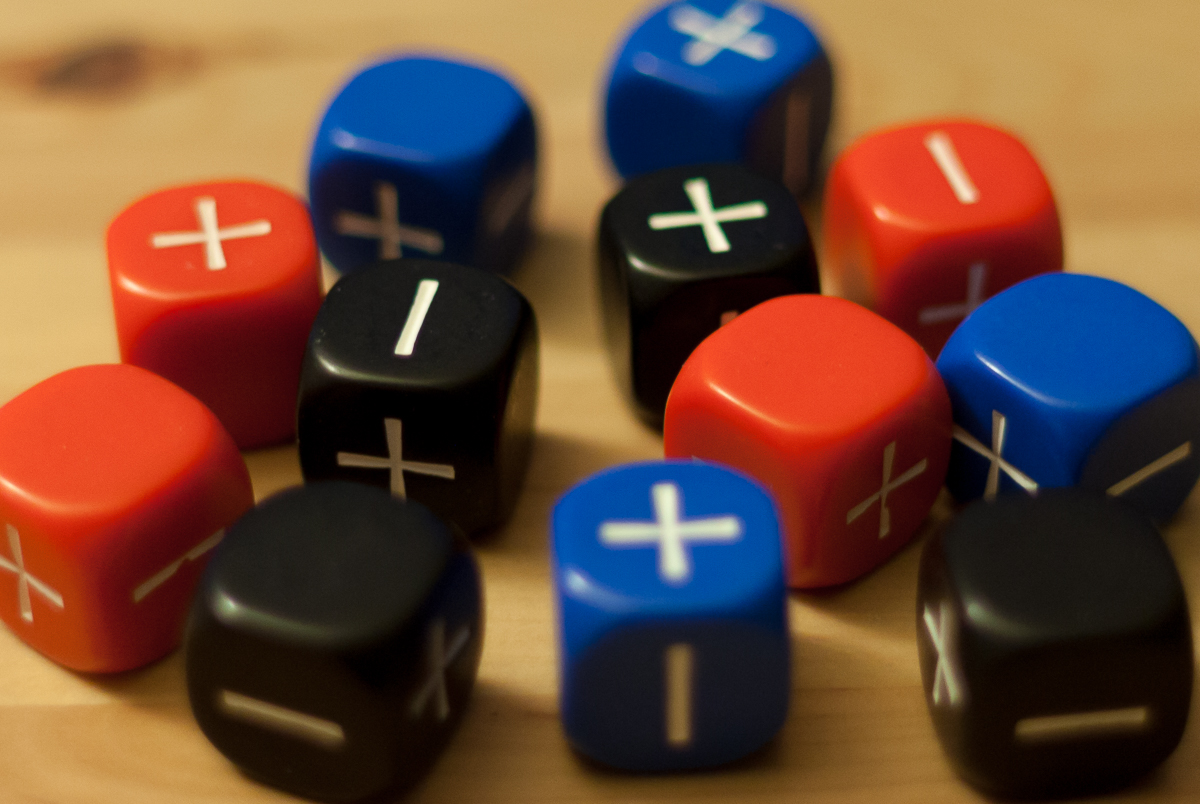# Fate Dice StatisticsMy friends and I played Fate, a role-playing game, for a few years during graduate school. Over that time we developed superstitions about the various dice we rolled. Since we were (are) huge nerds we decided to record (almost) all of the rolls to determine if the dice really were biased. We cursorily looked at the data when we finished playing, but I thought it would be interesting to dig it back out and analyze it more deeply.

## Fate Dice

Fate dice (also called Fudge dice) have six sides and three values with equal probability of appearing: plus, blank, and minus. These respectively represent +1, 0, and -1 . Four dice are rolled at a time and their results are summed, giving a range of -4 to 4. The notation for this type of roll is 4dF.

Figuring out the probability of rolling a value is just simple combinatorics. These probabilities are:

Value Probability
0 19/81
1 xor -1 16/81
2 xor -2 10/81
3 xor -3 4/81
4 xor -4 1/81

## Rolls

We had four sets of Fate dice, colored blue, red, black, and white. We wrote down only the sum of each roll, since the individual dice in the set are indistinguishable. This means that if one of the dice is biased, it will take longer to show up than if we had been able to explore the results individually. As per usual, you can find the Jupyter notebook used to make these calculations here (rendered on Github). The data is here.

Here are the distributions of rolls for each of the four sets of dice. The points indicate the number of rolls that came up with a certain value, while the grey area is the range in which we would expect to find a result produced by a fair set of dice 99% of the time. I discuss how these regions are computing in detail in another post.The blue dice were rolled the most (because we thought the red and black sets were unlucky), but visual inspection suggests that they were actually biased! Contrary to our superstitions, the “cursed” red and black dice seem to have been fine. The white dice have one bin (very) high, but it’s hard to tell by eye if that is significant.

## Significance

To check whether the dice are biased, a chi-squared test is required. The chi-squared test essentially looks at how far away each point in a distribution is from the expected value for that point, and normalizes by the variance. The test statistic is then compared to the results expected from a chi-squared distribution and a significance is obtained. Running this test on our dice yields the following results:

Dice chi-squared p-value
Blue 26.31 0.001
Black 9.32 0.315
Red 10.77 0.215
White 19.07 0.014

The chi-squared test has some caveats about low expected values, but at worst we only have two (out of nine) bins below five expected entries. Looking at the p-values we conclude roughly the same as our “chi-by-eye” test above: the blue dice are significantly biased, while the black and red dice show no evidence of being unfair. The white dice are not biased at the p < 0.01 level, but that single high bin is odd and to be absolutely sure I would want to roll them a lot more and check.